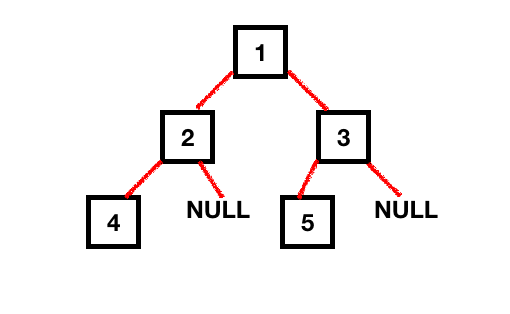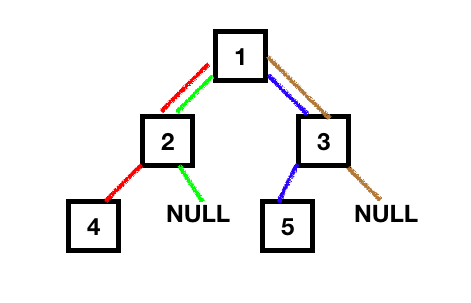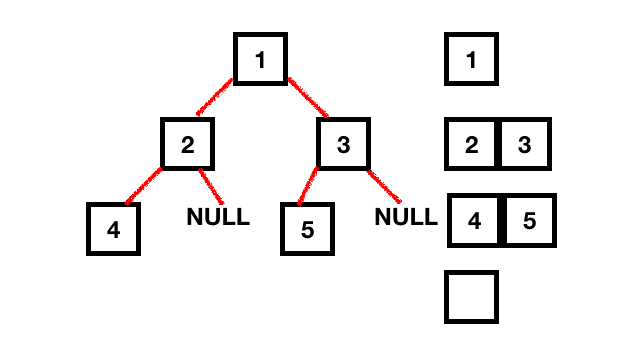# Height of a Tree Data Structure

Published on August 3, 2022By Anupam ChughWhile we believe that this content benefits our community, we have not yet thoroughly reviewed it. If you have any suggestions for improvements, please let us know by clicking the “report an issue“ button at the bottom of the tutorial.

In this tutorial, we’ll be discussing Binary Trees. We’ll see how to calculate the height of a tree data structure recursively as well as iteratively.

## Binary Trees

Binary Trees are a data structure in which data is stored in a hierarchical manner rather than linear (as it is done in LinkedList and Arrays). A Binary tree data structure consists of nodes. Each node holds the data along with the reference to the child pointers (left and right). The root of the binary tree is the topmost node. (So opposite of an actual living tree). Following is an illustration of a tree with some nodes.The height of a node is the length of the longest downward path to a leaf from that node. The height of the root is the height of the tree. So, in order to calculate the height of the tree, we need to go through each node of the tree in order to obtain all permutations and combinations. There are two ways to calculate the height of the tree.

• Recursive Way
• Iterative Way

### Height of a Tree - Recursively

Recursion involves calculating the results of the subproblems and returning it back to the parent problem.

Steps involved:

1. To calculate the height of the tree recursively, we need to find the height of it’s left subtree and right subtree recursively and add 1 to them (height between the topmost node and its children).
2. Each of these subtrees could have a left and right subtree themselves, hence recursion would apply until the subtrees are NULL. The height of a null tree node is -1.
3. Finally, we’ll compare the heights of the left and right subtree and return the one which is greater.

Following illustration shows the number of permutations to calculate the height of the binary tree.Let’s write the Java program to calculate the height of the tree recursively. First of all, we will have a basic implementation of the Tree data structure.

``````package com.journaldev.tree.height;

public class BinaryTree {

TreeNode root;

public static class TreeNode {

TreeNode left;
TreeNode right;
Object data;

TreeNode(Object data) {
this.data = data;
left = right = null;
}
}
}
``````

Let’s see the code for finding the height of the tree using recursion.

``````package com.journaldev.tree.height;

import com.journaldev.tree.height.BinaryTree.TreeNode;

public class HeightOfTree {

public static void main(String[] args) {

BinaryTree binaryTree = new BinaryTree();

/**
* Binary Tree in our example, height = 2
* 		1		(Root)
* 	  2	  3		(Level 1)
*  4    	 5		(Level 2)
*/
binaryTree.root = new TreeNode(1);
binaryTree.root.left = new TreeNode(2);
binaryTree.root.right = new TreeNode(3);
binaryTree.root.left.left = new TreeNode(4);
binaryTree.root.right.left = new TreeNode(5);

int heightOfTree = height(binaryTree.root);
System.out.printf("Height of tree is %d", heightOfTree);
}

public static int height(TreeNode root) {

if (root == null)
return -1;

int leftHeight = height(root.left);
int rightHeight = height(root.right);

return Math.max(leftHeight, rightHeight) + 1;
}
}
``````

So, in the above code, once we reach the bottom-most child node, we add one to the height of the tree and return the result to the previous call. Output: Height of tree is 2 Let’s now do the same thing non-recursively.

### Height of the Tree - Iteratively

To calculate the height of the tree iteratively, we simply need to calculate the number of levels in the tree.

Steps involved

1. Create a Queue and add the root of the tree to it.
2. Pop the node from the queue and traverse down the queue while adding the child nodes to the queue.
3. In each iteration pop, the latest element added to the queue and add the elements of the next level (of this element) to the queue.
4. Do this until the queue size becomes zero. That would mean that the next level has zero elements.
5. For every traversed level, add 1.

Following is the iterative program to calculate the height of the tree.

``````public static int heightIteratively(TreeNode root) {

if (root == null)
return -1;

int height = -1;

while (!queue.isEmpty()) {
int size = queue.size();

height++;

while (size > 0) {
TreeNode treeNode = queue.remove();

if (treeNode.left != null)

if (treeNode.right != null)

size--;
}
}
return height;
}
``````

The above code keeps running until the queue isn’t empty. And also, it keeps adding all the elements at the next level while removing the current level items from the queue.Time Complexity is O(n). Space Complexity is O(1).

You can checkout complete code and more DS & Algorithm examples from our GitHub Repository.

Thanks for learning with the DigitalOcean Community. Check out our offerings for compute, storage, networking, and managed databases.Anupam Chugh

author

#### Still looking for an answer?

Ask a questionSearch for more help

JournalDev
DigitalOcean EmployeeSeptember 20, 2021

COuld you please explain why there is a +1 in the recursive algorithm? Wouldn’t a leaf (with no children aka height 0) also return 1 even tho the height of that subtree would technically be 0?

- redbrivk

JournalDev
DigitalOcean EmployeeMarch 5, 2021

Only the main function needs to be public. I ran the code with this configuration and had no issues.

- Jaminix

JournalDev
DigitalOcean EmployeeJune 15, 2020

can u help me with recursion implementation of the question

- Sourav

Click below to sign up and get \$200 of credit to try our products over 60 days!

### Popular Topics##### Hollie's Hub for Good

Working on improving health and education, reducing inequality, and spurring economic growth? We’d like to help.##### Become a contributor

You get paid; we donate to tech nonprofits.

##### Welcome to the developer cloud

DigitalOcean makes it simple to launch in the cloud and scale up as you grow – whether you’re running one virtual machine or ten thousand.© 2023 DigitalOcean, LLC.